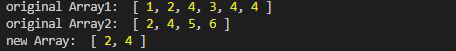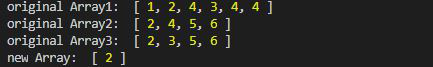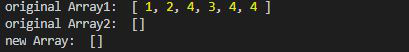Open In App

# Lodash _.Intersection() Method

Lodash is a JavaScript library that works on the top of underscore.js. Lodash helps in working with arrays, strings, objects, numbers etc.
The _.intersection() method is used to take the intersection of the one or more arrays. It is same as the intersection in set theory.

Syntax:

`_.intersection([arrays])`

Parameter: It takes an arrays as a parameter.

Return Value: It returns the array after intersection of arrays.

Note: Please install lodash module by using command `npm install lodash` before using the code given below.

Example 1: Taking Intersection of two arrays.

## Javascript

 `// Requiring the lodash library``const _ = require(``"lodash"``);`` ` `// Original array``let array1 = [1, 2, 4, 3, 4, 4]``let array2 = [2, 4, 5, 6]`` ` `// Using _.intersection() method``let newArray = lodash.intersection(``                ``array1, array2);`` ` `// Printing original Array``console.log(``"original Array1: "``, array1)``console.log(``"original Array2: "``, array2)`` ` `// Printing the newArray``console.log(``"new Array: "``, newArray)`

Output:Example 2: Taking intersection of more than two arrays.

## Javascript

 `// Requiring the lodash library``const _ = require(``"lodash"``);`` ` `// Original array``let array1 = [1, 2, 4, 3, 4, 4]``let array2 = [2, 4, 5, 6]``let array3 = [2, 3, 5, 6]`` ` `// Using _.intersection() method``let newArray = _.intersection(``        ``array1, array2, array3);`` ` `// Printing original Array``console.log(``"original Array1: "``, array1)``console.log(``"original Array2: "``, array2)``console.log(``"original Array3: "``, array3)`` ` `// Printing the newArray``console.log(``"new Array: "``, newArray)`

Output:Example 3: Intersection of array with the empty array.

## Javascript

 `// Requiring the lodash library``const _ = require(``"lodash"``);`` ` `// Original array``let array1 = [1, 2, 4, 3, 4, 4]``let array2 = []`` ` `// Using _.intersection() method``let newArray = lodash``    ``.intersection(array1, array2);`` ` `// Printing original Array``console.log(``"original Array1: "``, array1)``console.log(``"original Array2: "``, array2)`` ` `// Printing the newArray``console.log(``"new Array: "``, newArray) `

Output: